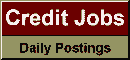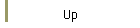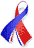DefaultRisk.com the web's biggest credit risk modeling resource.doi> search: A or B Export citation to:- HTML- Text (plain)- BibTeX- RIS- ReDIF

A Rating-based Model for Credit Derivatives

by Raphael Douady of RiskData, and
Monique Jeanblanc of Evry University

2002

Abstract: We present a model in which a bond issuer subject to possible default is assigned a "continuous" rating Rt Î[0,1] that follows a jump-diffusion process. Default occurs when the rating reaches 0, which is an absorbing state. An issuer that never defaults has rating 1 (unreachable). The value of a bond is the sum of "default-zero-coupon" bonds (DZC), prices as follows:

D(t,x,R)=exp(- (t,x)-y(t,x,R))

The default-free yield  y(t,x,1)= (t,x)/x follows a traditional interest rate model (e.g. HJM, BGM, "string", etc.). The "spread field" ψ(t,x,R) is a positive random function of two variables R and x, decreasing with respect to R and such that ψ(t,0,R=0). The value  ψ(t,x,0) is given by the bond recovery value upon default. The dynamics of ψ is represented as the solution of a finite dimensional SDE. Given ψ such that ∂ψ/∂R<0 a.s., we compute what should be the drift of the rating process Rt under the risk-neutral probability, assuming its volatility and possible jumps are also given.

For several bonds, ratings are driven by correlated Brownian motions and jumps are produced by a combination of economic events.

Credit derivatives are priced by Monte-Carlo simulation. Hedge ratios are computed with respect to underlying bonds and CDS's.

Most other credit models (Merton, Jarrow-Turnbull, Duffie-Singleton, Hull-White, etc.) can be seen either as particular cases or as limit cases of this model, which has been specially designed to ease calibration.

Long-term statistics on yield spreads in rating and seniority category provide the diffusion factors of ψ. The rating process is, in a first step, statistically estimated, thanks to agency rating migration statistics from rating agencies (each agency rating is associated with a range for the continuous rating). Then its drift is replaced by the risk-neutral value, while the historical volatility and the jumps are left untouched.

Published in: European Investment Review, Vol. 1, (2002), pp. 17-29.

Books Referenced in this paper:  (what is this?)